## Topic 6.3: Transformations to Linear Regression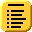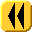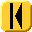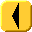Introduction Notes Theory HOWTO Examples Engineering Error Questions Matlab Maple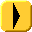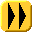Not all data may be represented by functions in the form y = c1f1(x) + ... + cnfn(x), for example, many responses are exponential in nature, that is the data follows a curve of the form y = c1ec2x which is not in the desired form for linear regression.

We want to be able to transform the exponential function into a linear sum of functions. Here we will look at some transformations which may be used to convert such data so that we may use the least squares method to find the best fitting curve.

Note: Matlab uses the log function to calculate the natural logarithm, and therefore in these notes, we will use log(x) to calculate what you would normally write as ln(x) in your calculus course.